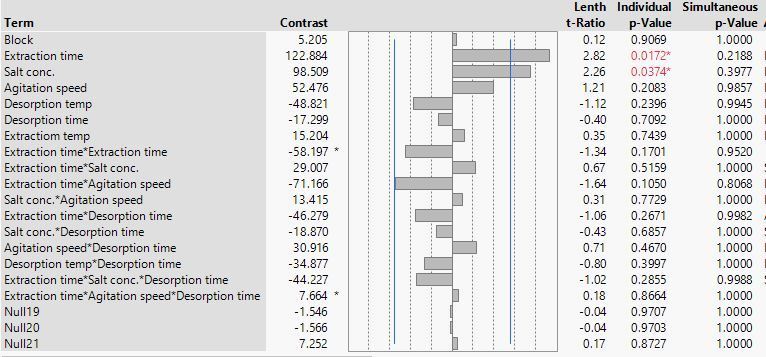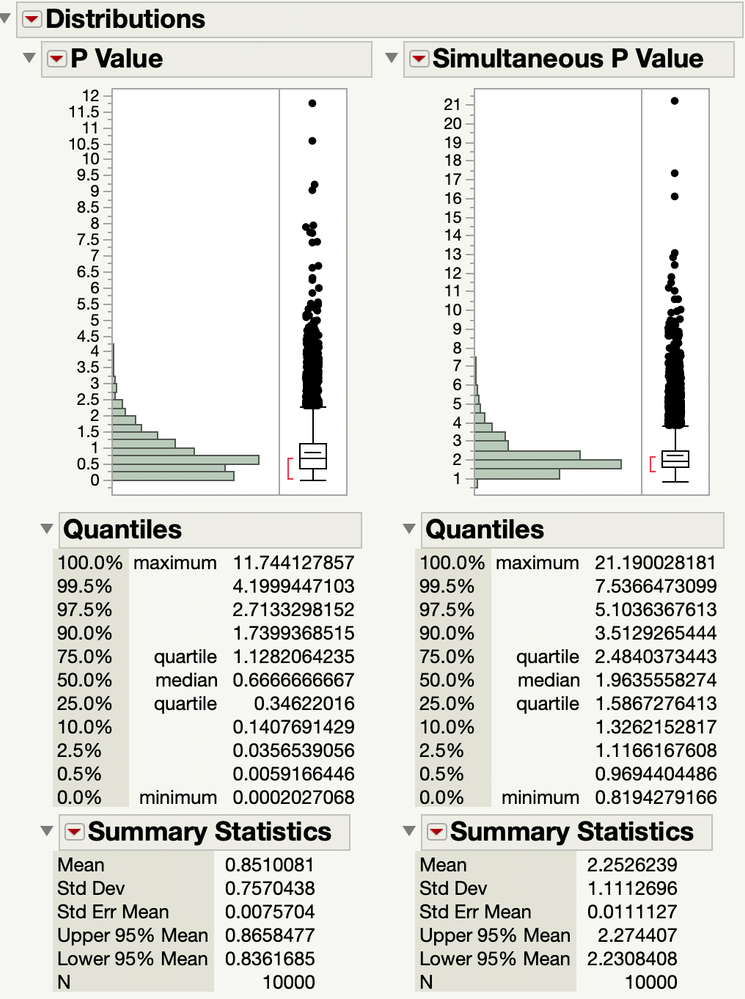Our World Statistics Day conversations have been a great reminder of how much statistics can inform our lives. Do you have an example of how statistics has made a difference in your life? Share your story with the Community!
Choose Language Hide Translation Bar
Highlighted

## How to determine the Length t-ratio corresponding to p-value of 0.05 from screening design?

Hi,

I am a new user of JMP12.

I am having a hard time to interpret the results of a screening design (please see the attached screenshot). I know JMP makes this split-plot by showing the Lenth t-ratio of each effect as the length of each bar. Also, the blue vertical line indicates the effects with individual p-value of 0.1.

Now, I was wondering if there is any way to know the Length t-ratio corresponding to an individual p-value of 0.05 from this result.

Thanks :)5 REPLIES 5
Highlighted

## Re: How to determine the Length t-ratio corresponding to p-value of 0.05 from screening design?

The Lenth t-ratio does not have a parametric distribution model. The p-values and simultaneous p-values in the Screening platform are computed by 10,000 Monte Carlo simulations of the Lenth t-ratio under the null hypothesis to provide an empirical sampling distribution of the statistics. (So the values that you see will vary each time you run the Screening platform on the same data.) The empirical distribution depends on the number of contrasts in the Screening platform, which is always equal to the number of runs.

Here is the empirical distribution for a case with 15 contrasts:So the Quantiles report shows the absolute value of the t-ratios.

Learn it once, use it forever!
Highlighted

## Re: How to determine the Length t-ratio corresponding to p-value of 0.05 from screening design?

Hi Markbailey,

Thanks a lot for the clarification. So what I understand is, JMP does 10,000 simulations and based on the simulated t-ratio it computed the p-values. But how does it actually compute the p-values? I mean, does it use the t-distribution table to calculate the p-values from the degrees of freedom and simulated t-ratios? I am just trying to get an idea about how actually JMP comes up with all these values.

Thanks again!

Highlighted

## Re: How to determine the Length t-ratio corresponding to p-value of 0.05 from screening design?

The standard approach in bootstrapping is to sort the simulated statistics, then find the position of your sample statistic in the list. If 90% of the statistics were less than your estimate, the p-value is 0.1.

That is exactly the same as finding the p-value for a sample t statistic with a parametric t-distribution.

Learn it once, use it forever!
Highlighted

## Re: How to determine the Length t-ratio corresponding to p-value of 0.05 from screening design?

Thanks again!

Just for clarification, JMP by default uses the two-tailed t-test, right?

So, for example, if my screening design matrix has a degree of freedom 15, can I use the simulated t-ratios from JMP to manually calculate the p-values from a standard two-tailed t-distribution table? Will my calculated p-value will match with that from the JMP? I understand my calculated p-values might not be exactly the same, but is this a valid approach based on the algorithm JMP uses?

Highlighted

## Re: How to determine the Length t-ratio corresponding to p-value of 0.05 from screening design?

Yes, the Screening platform uses a two-sided t test with Lenth's PSE for the denominator.

Yes, bootstrapping sample statistics was established in the 1970s as a valid non-parametric approach to inference.

No, you cannot use the parametric t distribution to obtain the p-values for the Lenth t ratio. That is the whole point of using the Monte Carlo approach. It would be much easier to use the distribution model but the p values would not be accurate.

Learn it once, use it forever!
Article Labels

There are no labels assigned to this post.